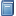Buchempfehlung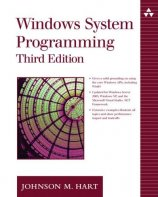Windows System Programming
Das Kompendium liefert viele interessante Informationen zur Windows-Programmierung auf Englisch. [Mehr Infos...]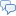FreeBASIC-Chat
Es sind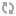Benutzer im FreeBASIC-Chat online.
(Stand:  )

# fb:porticula NoPaste

## FB2HTML.bas

 Uploader: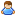ThePuppetMaster Datum/Zeit: 24.01.2008 05:56:46
Hinweis: Dieser Quelltext ist Bestandteil des Projekts FB2HTML, zu dem es auf FreeBASIC-Portal.de eine Projektseite gibt.

```'############################################################################################################## '   F B 2 H T M L '############################################################################################################## 'Autor:  Martin Wiemann 'Create: 22.01.2008 '############################################################################################################## #IF DEFINED(__FB_LINUX__)     Const G_Seperator = "/" #ELSEIF DEFINED(__FB_WIN32__)     Const G_Seperator = "\" #ELSE     #error "Unsupported platform" #ENDIF '############################################################################################################## Declare Sub         Main        () Declare Function    InStrRev    (ByVal V_Data as String, V_Search as String) as Long Declare Function    MakeWord    (V_Data as String) as String Declare Function    F_CheckHex  (V_Data as String) as UByte '############################################################################################################## Main() End 0 '############################################################################################################## Sub Main() Dim T       as String Dim T1      as String Dim X       as ULong Dim Y       as ULong Dim XPos    as ULong Dim DD()    as String Dim DC      as ULong Dim XABSC   as ULong Dim XPathFileIn             as String Dim XPathFileOut            as String Dim XLineNum                as UByte = 1 Dim XColorBack              as String = "FFFFFF" Dim XColorFore              as String = "000000" Dim XColorComment           as String = "008800" Dim XColorString            as String = "FF0000" Dim XColorCommand           as String = "0000FF" Dim XColorStatement         as String = "6666FF" Dim XColorDatatype          as String = "AA5500" Dim XColorPreProcStatement  as String = "00AAAA" Dim XColorPreProcVar        as String = "AA00AA" T = Command For X = 1 to Len(T)     XPos = InStr(1, T, " ")     If XPos > 0 Then         T1 = Mid(T, 1, XPos - 1): T = Mid(T, XPos + 1)     Else: T1 = T: T = ""     End If     If T1 <> "" Then         DC += 1: Redim Preserve DD(DC) as String         DD(DC) = T1     End If     If T = "" Then Exit For Next Select Case DC     case 0: Print "Missing commands! Use --help to show commands!": End -1     case 1         Select case lcase(DD(1))             case "--help", "-help"                 Print ""                 Print "   #####################################################"                 Print "  ### freeBASIC sourcecode to HTML-script converter ###"                 Print " #####################################################"                 Print ""                 Print ""                 Print "Syntax:  fb2html <command / path> [<option>]"                 Print ""                 Print ""                 Print "example: fb2html incode.bas"                 Print "   Will read file 'incode.bas' and create html in new incode.html"                 Print ""                 Print "example: fb2html -i incode.bas -o outcode.html -cb FF00FF -cf 00FF00"                 Print "   Will read file 'incode.bas' and create html in new outcode.html"                 Print "   New HTML code will colorized whis FF00FF as Backcolor and 00FF00 als Forecolor"                 Print ""                 Print ""                 Print ""                 Print "-!- .bas files was convert to to .html"                 Print "-!- .bi files was convert to to .htm"                 Print "-!- other files will only be convert whis -i and -o option!"                 Print ""                 Print ""                 Print "  --help / -help       = Show help text. (This text)"                 Print ""                 Print "  -i <path/file>       = Input path / file"                 Print "  -o <path/file>       = Output path / file"                 Print ""                 Print "  -noline              = will toggel the visibility of the line numbers"                 Print ""                 Print "  -cb <hex-color>      = Color value for background like 000000 to FFFFFF"                 Print "  -cf <hex-color>      = Foreground color (regulare text)"                 Print "  -cs <hex-color>      = String color (text in " & chr(34,34) & " tags)"                 Print "  -cc <hex-color>      = Comments color (text after ' tag)"                 Print "  -co <hex-color>      = Basecommand color (text like 'Print')"                 Print "  -cm <hex-color>      = Statement color (text like 'byval')"                 Print "  -cd <hex-color>      = Datatype color (text like 'Long')"                 Print "  -cps <hex-color>     = Preporcessor Statement color (text like '#IFDEF')"                 Print "  -cpv <hex-color>     = Preporcessor Variable color (text like '__fb_linux__')"                 Print ""                 end 0             case else: XPathFileIn  = DD(1)         end Select     case else         For X = 1 To DC             Select case lcase(DD(X))                 case "-i"                     If DC <= X Then Print "Missing path / file!": End -1                     X += 1: XPathFileIn = DD(X)                 case "-o"                     If DC <= X Then Print "Missing path / file!": End -1                     X += 1: XPathFileOut = DD(X)                 case "-noline": XLineNum = 0                 case else                     If DC <= X Then Print "Missing hex value!": End -1                     If F_CheckHex(DD(X + 1)) = 0 Then Print "'" & DD(X + 1) & "' is not a hex value": End -1                     X += 1                     Select Case lcase(DD(X - 1))                         case "-cb": XColorBack              = lcase(DD(X))                         case "-cf": XColorFore              = lcase(DD(X))                         case "-cs": XColorString            = lcase(DD(X))                         case "-cc": XColorCommand           = lcase(DD(X))                         case "-cm": XColorStatement         = lcase(DD(X))                         case "-cd": XColorDatatype          = lcase(DD(X))                         case "-cps": XColorPreProcStatement = lcase(DD(X))                         case "-cpv": XColorPreProcVar       = lcase(DD(X))                         case else: Print "Unknow commands! Use --help to show commands! [" & lcase(DD(X - 1)) & "]": End -1                     End Select             end Select         Next End Select If XPathFileIn = "" Then Print "Inputfile was not found!": End -1 If Dir(XPathFileIn) = "" Then Print "Inputfile was not found!": End -1 Dim XInPath     as String Dim XInName     as String Dim XInType     as String Dim XOutPath    as String Dim XOutName    as String Dim XOutType    as String XPos = InStrRev(XPathFileIn, G_Seperator) If XPos > 0 Then     XInName = Mid(XPathFileIn, XPos + 1)     XInPath = Mid(XPathFileIn, 1, XPos) Else: XInName = XPathFileIn End If If XPathFileOut <> "" Then     XPos = InStrRev(XPathFileOut, G_Seperator)     If XPos > 0 Then         XOutName = Mid(XPathFileOut, XPos + 1)         XOutPath = Mid(XPathFileOut, 1, XPos)     Else: XOutName = XPathFileOut     End If End IF If XOutPath <> "" Then If Right(XOutPath, 1) <> G_Seperator Then Print "Outputpath corrupt!": End -1 XPos = InStrRev(XInName, ".") If XPos = 0 Then Print "can't find input filetype! (.bas / .bi / .*)": End -1 XInType = Mid(XInName, XPos + 1) XInName = Mid(XInName, 1, XPos - 1) If XOutName <> "" Then     XPos = InStrRev(XOutName, ".")     If XPos = 0 Then Print "can't find output filetype! (.html / .htm)": End -1     XOutType = Mid(XOutName, XPos + 1)     XOutName = Mid(XOutName, 1, XPos - 1)     Select case lcase(XOutType)         case "html", "htm"         case else: Print "Output filetype was not supported by converter.": End -1     End Select Else     XOutName = XInName     Select case LCase(XInType)         case "bas": XOutType = "html"         case "bi": XOutType = "htm"         case else: Print "-o command need for .* filetype": End -1     End Select End If Dim G_FNIn          as Integer Dim G_FNOut         as Integer Dim TOutD()         as String Dim TOutC           as ULong = 1 Redim TOutD(TOutC)  as String Dim FBCRLF          as String = Chr(13,10) If Dir(XOutPath & XOutName & "." & XOutType) <> "" Then Kill XOutPath & XOutName & "." & XOutType G_FNOut = FreeFile Open XOutPath & XOutName & "." & XOutType for binary as #G_FNOut Print #G_FNOut, "<!DOCTYPE HTML PUBLIC ""-//W3C//DTD HTML 4.01 Transitional//EN"">" Print #G_FNOut, "<html>" Print #G_FNOut, " <head>" Print #G_FNOut, "  <title>Freebasic-Sourcecode of: '" & XInName & "." & XInType & "'</title>" Print #G_FNOut, "  <style type=""text/css"">" Print #G_FNOut, "   body{font-family:lucida console,fixedsys,monospace;font-size:11px;line-height:12px;}" Print #G_FNOut, "   .ca{color:#" & XColorCommand & ";font-weight:bold;}" Print #G_FNOut, "   .cb{color:#" & XColorStatement & ";font-weight:bold;}" Print #G_FNOut, "   .cc{color:#" & XColorDatatype & ";}" Print #G_FNOut, "   .cd{color:#" & XColorPreProcStatement & ";}" Print #G_FNOut, "   .ce{color:#" & XColorPreProcVar & ";}" Print #G_FNOut, "   .cf{color:#" & XColorComment & ";font-style:italic;}" Print #G_FNOut, "   .cg{color:#" & XColorString & ";}" Print #G_FNOut, "   .cz{color:#" & XColorFore & ";font-family:lucida console,fixedsys,monospace;font-size:12px;line-height:12px;float:left;text-align:right;padding-left:10px;padding-right:10px;}" Print #G_FNOut, "   .cy{color:#" & XColorFore & ";font-family:lucida console,fixedsys,monospace;font-size:12px;line-height:12px;float:left;text-align:left;padding-left:10px;padding-right:10px;}" Print #G_FNOut, "  </style>" Print #G_FNOut, " </head>" Print #G_FNOut, " <body bgcolor=""#" & XColorBack & """ text=""#" & XColorFore & """ link=""#" & XColorFore & """ vlink=""#" & XColorFore & """ alink=""#" & XColorFore & """>" G_FNIn = FreeFile Open XPathFileIn for binary as #G_FNIn Dim XLen        as ULong = Lof(G_FNIn) Dim XALock      as UByte Dim XLLock      as UByte Dim XWCaS       as String Dim XTS         as ULong For Y = 1 to XLen step 1024     T = Space(1024)     If Y + 1024 > XLen Then T = Space(XLen - Y + 1)     Get #G_FNIn, Y, T     For X = 1 to Len(T)         Select Case Mid(T, X, 1)             case """"                 If XLLock = 0 Then                     XABSC += Len(XWCaS) + 1                     If XALock = 0 Then                         TOutD(TOutC) += MakeWord(XWCaS): XWCaS = ""                         XALock = 1: TOutD(TOutC) += "<span class=""cg"">""                     Else                         TOutD(TOutC) += XWCaS: XWCaS = ""                         XALock = 0: TOutD(TOutC) += ""</span>"                     End If                 Else: TOutD(TOutC) += """: XABSC += 1                 End If                              case ":"                 XABSC += Len(XWCaS) + 1                 If (XALock = 0) and (XLLock = 0) Then                     TOutD(TOutC) += MakeWord(XWCaS): XWCaS = ""                 Else: TOutD(TOutC) += XWCaS: XWCaS = ""                 End If                 TOutD(TOutC) += ":"             case chr(9)                 XABSC += Len(XWCaS)                 XTS = 4 - (XABSC mod 4)                 If (XALock = 0) and (XLLock = 0) Then                     TOutD(TOutC) += MakeWord(XWCaS) & String(XTS, " "): XWCaS = ""                 Else: TOutD(TOutC) += XWCaS & String(XTS, " "): XWCaS = ""                 End If                 XABSC += XTS             case chr(13)             case chr(10)                 If (XALock = 0) and (XLLock = 0) Then                     TOutD(TOutC) += MakeWord(XWCaS): XWCaS = ""                 Else: TOutD(TOutC) += XWCaS: XWCaS = ""                 End If                 If XLLock = 1 Then TOutD(TOutC) += "</span>"                 If XALock = 1 Then TOutD(TOutC) += "</span>"                 TOutD(TOutC) += Chr(13,10)                 TOutC += 1                 Redim Preserve TOutD(TOutC) as String                 XLLock = 0                 XALock = 0                 XABSC = 0             case " ", "<", ">", "&", "(", ")", ","                 XABSC += Len(XWCaS) + 1                 Select case Mid(T, X, 1)                     case " ": T1 = " "                     case "<": T1 = "<"                     case ">": T1 = ">"                     case "&": T1 = "&"                     case Else: T1 = Mid(T, X, 1)                 End Select                 If (XALock = 0) and (XLLock = 0) Then                     TOutD(TOutC) += MakeWord(XWCaS) & T1: XWCaS = ""                 Else: TOutD(TOutC) += XWCaS & T1: XWCaS = ""                 End If             case "'"                 XABSC += Len(XWCaS) + 1                 If XALock = 0 Then                     TOutD(TOutC) += MakeWord(XWCaS): XWCaS = ""                     If XLLock = 0 Then TOutD(TOutC) += "<span class=""cf"">"                     XLLock = 1                 Else: TOutD(TOutC) += XWCaS: XWCaS = ""                 End If                 TOutD(TOutC) += Mid(T, X, 1)             case else: XWCaS += Mid(T, X, 1)         End Select     Next Next Close #G_FNIn TOutD(TOutC) += MakeWord(XWCaS) Print #G_FNOut, "  <table border=""0"" cellpadding=""1"" cellspacing=""2"">" Print #G_FNOut, "   <tr>" If XLineNum = 1 Then     Print #G_FNOut, "    <td class=""cz"" align=""right"" valign=""top"">"     For X = 1 To TOutC         Print #G_FNOut, str(x) & ":<br>";     Next     Print #G_FNOut, "    </td>" End If Print #G_FNOut, "    <td class=""cy"" align=""left"" valign=""top"">" Print #G_FNOut, "     <pre>"; For X = 1 To TOutC     Print #G_FNOut, TOutD(X); Next Print #G_FNOut, "     </pre>" Print #G_FNOut, "    </td>" Print #G_FNOut, "   </tr>" Print #G_FNOut, "  </table>" Print #G_FNOut, " </body>" Print #G_FNOut, "</html>" Close #G_FNOut Print "convert successful!" End Sub '############################################################################################################## Function InStrRev(ByVal V_Data as String, V_Search as String) as Long Dim X   as Long Dim SL  as Long SL = Len(V_Search) For X = Len(V_Data) - SL + 1 to 1 Step - 1     If Mid(V_Data, X, SL) = V_Search then Return X Next End Function '############################################################################################################## Function MakeWord(V_Data as String) as String Dim XT  as UByte select case LCase(left(V_Data, 1))     case "_"         Select Case LCase(V_Data)             case "__date__":                XT = 4             case "__fb_argc":               XT = 4             case "__fb_argv__":             XT = 4             case "__fb_bigendian__":        XT = 4             case "__fb_debug__":            XT = 4             case "__fb_dos__":              XT = 4             case "__fb_err__":              XT = 4             case "__fb_lang__":             XT = 4             case "__fb_linux__":            XT = 4             case "__fb_main__":             XT = 4             case "__fb_min_version__":      XT = 4             case "__fb_mt__":               XT = 4             case "__fb_option_byval__":     XT = 4             case "__fb_option_dynamic__":   XT = 4             case "__fb_option_escape__":    XT = 4             case "__fb_option_explicit__":  XT = 4             case "__fb_option_private__":   XT = 4             case "__fb_out_dll__":          XT = 4             case "__fb_out_exe__":          XT = 4             case "__fb_out_lib__":          XT = 4             case "__fb_out_obj__":          XT = 4             case "__fb_signature__":        XT = 4             case "__fb_ver_major__":        XT = 4             case "__fb_ver_minor__":        XT = 4             case "__fb_ver_patch__":        XT = 4             case "__fb_version__":          XT = 4             case "__fb_win32__":            XT = 4             case "__file__":                XT = 4             case "__file_nq__":             XT = 4             case "__function__":            XT = 4             case "__line__":                XT = 4             case "__path__":                XT = 4             case "__time__":                XT = 4         end select     case "#"         Select Case LCase(V_Data)             case "#if":             XT = 5             case "#ifdef":          XT = 5             case "#elseif":         XT = 5             case "#else":           XT = 5             case "#endif":          XT = 5             case "#ifndef":         XT = 5             case "#error":          XT = 5         End Select              case "a"         Select Case LCase(V_Data)             case "abs":             XT = 1             case "access":          XT =    2             case "acos":            XT = 1             case "add":             XT =    2             case "alias":           XT =    2             case "allocate":        XT = 1             case "alpha":           XT = 1             case "and":             XT =    2             case "any":             XT =        3             case "append":          XT =    2             case "as":              XT =    2             case "asc":             XT = 1             case "asin":            XT = 1             case "asim":            XT = 1             case "asm":             XT =    2             case "assert":          XT =    2             case "assertwarn":      XT =    2             case "atan2":           XT = 1             case "atn":             XT = 1         end select     case "b"         Select Case LCase(V_Data)             case "base":            XT =    2             case "beep":            XT =    2             case "bin":             XT = 1             case "binary":          XT =    2             case "bit":             XT = 1             case "bitreset":        XT = 1             case "bitset":          XT = 1             case "bload":           XT = 1             case "bsave":           XT = 1             case "byte":            XT =        3             case "byref":           XT =    2             case "byval":           XT =    2         end select     case "c"         Select Case LCase(V_Data)             case "call":            XT =    2             case "callocate":       XT = 1             case "calls":           XT =    2             case "case":            XT = 1             case "cast":            XT = 1             case "cbyte":           XT = 1             case "cdbl":            XT = 1             case "cdecl":           XT =    2             case "chain":           XT = 1             case "chdir":           XT = 1             case "chr":             XT = 1             case "cint":            XT = 1             case "circel":          XT =    2             case "clear":           XT =    2             case "clng":            XT = 1             case "clngint":         XT = 1             case "close":           XT =    2             case "cls":             XT =    2             case "color":           XT =    2             case "com":             XT =    2             case "command":         XT = 1             case "common":          XT =    2             case "condbroadcast":   XT = 1             case "condcreate":      XT = 1             case "conddestroy":     XT = 1             case "condsignal":      XT = 1             case "condwait":        XT = 1             case "cons":            XT =    2             case "const":           XT =    2             case "constructor":     XT =    2             case "continue":        XT =    2             case "cos":             XT = 1             case "cptr":            XT = 1             case "cshort":          XT = 1             case "csign":           XT = 1             case "csng":            XT = 1             case "csrlin":          XT = 1             case "cubyte":          XT = 1             case "cuint":           XT = 1             case "culngint":        XT = 1             case "cunsg":           XT = 1             case "curdir":          XT = 1             case "cushort":         XT = 1             case "custom":          XT =    2             case "cvd":             XT = 1             case "cvi":             XT = 1             case "cvl":             XT = 1             case "cvlongint":       XT = 1             case "cvs":             XT = 1             case "cvshort":         XT = 1         end select     case "d"         Select Case LCase(V_Data)             case "data":            XT =    2             case "date":            XT = 1             case "dateadd":         XT = 1             case "datediff":        XT = 1             case "datepart":        XT = 1             case "dateserial":      XT = 1             case "datevalue":       XT = 1             case "day":             XT = 1             case "deallocate":      XT = 1             case "declare":         XT = 1             case "defbyte":         XT =    2             case "defdbl":          XT =    2             case "define":          XT =    2             case "defined":         XT = 1             case "defint":          XT =    2             case "deflng":          XT =    2             case "deflongint":      XT =    2             case "defshort":        XT =    2             case "defsng":          XT =    2             case "defstr":          XT =    2             case "defubyte":        XT =    2             case "defuint":         XT =    2             case "defulongint":     XT =    2             case "defushort":       XT =    2             case "delete":          XT =    2             case "destructor":      XT =    2             case "dim":             XT = 1             case "dir":             XT = 1             case "do":              XT = 1             case "double":          XT =        3             case "draw":            XT = 1             case "dylibfree":       XT = 1             case "dylibload":       XT = 1             case "dylibsymbol":     XT = 1             case "dynamic":         XT =    2         end select     case "e"         Select Case LCase(V_Data)             case "else":            XT = 1             case "elseif":          XT = 1             case "encoding":        XT = 1             case "end":             XT = 1             case "endif":           XT = 1             case "endmacro":        XT =    2             case "enum":            XT = 1             case "environ":         XT =    2             case "eof":             XT = 1             case "eqv":             XT = 1             case "erase":           XT =    2             case "erfn":            XT = 1             case "ermn":            XT = 1             case "err":             XT =    2             case "error":           XT =    2             case "escape":          XT =    2             case "exec":            XT = 1             case "exepath":         XT = 1             case "exit":            XT = 1             case "exp":             XT = 1             case "explicite":       XT =    2             case "export":          XT =    2             case "extern":          XT =    2         end select     case "f"         Select Case LCase(V_Data)             case "field":           XT = 1             case "fileattr":        XT = 1             case "filecopy":        XT = 1             case "filedatetime":    XT = 1             case "fileexist":       XT = 1             case "filelen":         XT = 1             case "fix":             XT = 1             case "flip":            XT = 1             case "for":             XT = 1             case "format":          XT = 1             case "frac":            XT = 1             case "fre":             XT = 1             case "freefile":        XT = 1             case "function":        XT = 1         end select     case "g"         Select Case LCase(V_Data)             case "get":             XT = 1             case "getjoystick":     XT = 1             case "getkey":          XT = 1             case "getmouse":        XT = 1             case "gosub":           XT =    2             case "goto":            XT =    2         end select     case "h"         Select Case LCase(V_Data)             case "hex":             XT = 1             case "hibyte":          XT = 1             case "hiword":          XT = 1             case "hour":            XT = 1         end select     case "i"         Select Case LCase(V_Data)             case "if":              XT = 1             case "ifdef":           XT =    2             case "ifndef":          XT =    2             case "iif":             XT = 1             case "imageconvertrow": XT = 1             case "imagecreate":     XT = 1             case "imagedestroy":    XT = 1             case "imp":             XT =    2             case "import":          XT = 1             case "inclib":          XT = 1             case "include":         XT = 1             case "inkey":           XT = 1             case "inp":             XT =    2             case "input":           XT =    2             case "instr":           XT = 1             case "instrrev":        XT = 1             case "int":             XT = 1             case "integer":         XT =        3             case "is":              XT = 1             case "isdate":          XT = 1         end select     case "k"         Select Case LCase(V_Data)             case "kill": XT = 1         end select     case "l"         Select Case LCase(V_Data)             case "lbound":          XT = 1             case "lcase":           XT = 1             case "left":            XT = 1             case "len":             XT = 1             case "let":             XT = 1             case "lib":             XT =    2             case "libpath":         XT =    2             case "line":            XT =    2             case "lobyte":          XT = 1             case "loc":             XT = 1             case "local":           XT = 1             case "locate":          XT = 1             case "lock":            XT = 1             case "lof":             XT = 1             case "loop":            XT = 1             case "log":             XT = 1             case "long":            XT =        3             case "longint":         XT =        3             case "loword":          XT = 1             case "lpos":            XT = 1             case "lprint":          XT = 1             case "lset":            XT = 1             case "ltrim":           XT = 1             case "lpt":             XT = 1         end select     case "m"         Select Case LCase(V_Data)             case "macro":           XT =    2             case "mid":             XT = 1             case "minute":          XT = 1             case "mkd":             XT = 1             case "mkdir":           XT = 1             case "mki":             XT = 1             case "mkl":             XT = 1             case "mklongint":       XT = 1             case "mks":             XT = 1             case "mkshort":         XT = 1             case "mod":             XT =    2             case "month":           XT = 1             case "monthname":       XT = 1             case "multikey":        XT = 1             case "mutexcreate":     XT = 1             case "mutexdestroy":    XT = 1             case "mutexlock":       XT = 1             case "mutexunlock":     XT = 1         end select     case "n"         Select Case LCase(V_Data)             case "name":            XT =    2             case "namespace":       XT =    2             case "next":            XT = 1             case "new":             XT =    2             case "not":             XT =    2             case "now":             XT = 1         end select     case "o"         Select Case LCase(V_Data)             case "option":          XT =    2             case "oct":             XT = 1             case "offsetop":        XT =    2             case "on":              XT =    2             case "once":            XT =    2             case "open":            XT = 1             case "operator":        XT =    2             case "or":              XT =    2             case "out":             XT =    2             case "output":          XT = 1             case "overload":        XT =    2         end select     case "p"         Select Case LCase(V_Data)             case "paint":           XT = 1             case "palette":         XT = 1             case "pascal":          XT = 1             case "pcopy":           XT = 1             case "peek":            XT =    2             case "pipe":            XT =    2             case "pmap":            XT = 1             case "point":           XT = 1             case "pointer":         XT = 1             case "poke":            XT =    2             case "pos":             XT = 1             case "pragma":          XT =    2             case "preserve":        XT =    2             case "preset":          XT =    2             case "print":           XT = 1             case "private":         XT =    2             case "procptr":         XT = 1             case "property":        XT =    2             case "pset":            XT = 1             case "ptr":             XT =    2             case "public":          XT =    2             case "put":             XT = 1         end select     case "r"         Select Case LCase(V_Data)             case "random":          XT =    2             case "randomize":       XT = 1             case "read":            XT = 1             case "reallocate":      XT = 1             case "redim":           XT =    2             case "rem":             XT =    2             case "reset":           XT = 1             case "restore":         XT =    2             case "resume":          XT = 1             case "return":          XT = 1             case "rgb":             XT = 1             case "rgba":            XT = 1             case "right":           XT = 1             case "rmdir":           XT = 1             case "rnd":             XT = 1             case "rset":            XT = 1             case "rtrim":           XT = 1             case "run":             XT = 1         end select     case "s"         Select Case LCase(V_Data)             case "sadd":            XT = 1             case "scope":           XT =    2             case "screen":          XT = 1             case "screencontrol":   XT = 1             case "screencopy":      XT = 1             case "screenevent":     XT = 1             case "screeninfo":      XT = 1             case "screenlist":      XT = 1             case "screenlock":      XT = 1             case "screenptr":       XT = 1             case "screenres":       XT = 1             case "screenset":       XT = 1             case "screensync":      XT = 1             case "screenunlock":    XT = 1             case "scrn":            XT = 1             case "second":          XT = 1             case "seek":            XT = 1             case "select":          XT = 1             case "setdate":         XT = 1             case "setenviron":      XT = 1             case "setmouse":        XT = 1             case "settime":         XT = 1             case "sgn":             XT = 1             case "shared":          XT =    2             case "shell":           XT = 1             case "shl":             XT = 1             case "short":           XT =        3             case "shr":             XT = 1             case "sin":             XT = 1             case "single":          XT =        3             case "sizeof":          XT = 1             case "sleep":           XT = 1             case "space":           XT = 1             case "spc":             XT = 1             case "sqr":             XT = 1             case "static":          XT =    2             case "stdcall":         XT = 1             case "step":            XT =    2             case "stop":            XT = 1             case "str":             XT = 1             case "string":          XT =        3             case "strptr":          XT = 1             case "sub":             XT = 1             case "system":          XT = 1         end select     case "t"         Select Case LCase(V_Data)             case "tab":             XT = 1             case "tan":             XT = 1             case "then":            XT = 1             case "this":            XT =    2             case "threadcreate":    XT = 1             case "threadwait":      XT = 1             case "time":            XT = 1             case "timer":           XT = 1             case "timeserial":      XT = 1             case "timevalue":       XT = 1             case "to":              XT =    2             case "trans":           XT = 1             case "trim":            XT = 1             case "type":            XT = 1         end select     case "u"         Select Case LCase(V_Data)             case "ubound":          XT = 1             case "ubyte":           XT =        3             case "ucase":           XT = 1             case "uinteger":        XT =        3             case "ulong":           XT =        3             case "ulongint":        XT =        3             case "undef":           XT =    2             case "union":           XT =    2             case "unlock":          XT =    2             case "unsigned":        XT =    2             case "using":           XT =    2             case "until":           XT = 1             case "ushort":          XT =        3         end select     case "v"         Select Case LCase(V_Data)             case "va_arg":          XT = 1             case "va_first":        XT = 1             case "va_next":         XT = 1             case "val":             XT = 1             case "val64":           XT = 1             case "valint":          XT = 1             case "vallng":          XT = 1             case "valuint":         XT = 1             case "valulng":         XT = 1             case "var":             XT = 1             case "varptr":          XT = 1             case "view":            XT = 1         end select     case "w"         Select Case LCase(V_Data)             case "wait":            XT = 1             case "wbin":            XT = 1             case "wchr":            XT = 1             case "weekday":         XT = 1             case "weekdayname":     XT = 1             case "whex":            XT = 1             case "while":           XT = 1             case "wend":            XT = 1             case "width":           XT = 1             case "window":          XT = 1             case "windowtitle":     XT = 1             case "winput":          XT = 1             case "with":            XT = 1             case "woct":            XT = 1             case "write":           XT = 1             case "wspace":          XT = 1             case "wstr":            XT = 1             case "wstring":         XT =        3         end select     case "x"         Select Case LCase(V_Data)             case "xor":             XT = 1         end select     case "y"         Select Case LCase(V_Data)             case "yeah":            XT = 1         end select     case "z"         Select Case LCase(V_Data)             case "zstring":         XT = 1         end select end select Select case XT     case 1: Return "<span class=""ca"">" & UCase(Left(V_Data, 1)) & LCase(Mid(V_Data, 2)) & "</span>"     case 2: Return "<span class=""cb"">" & UCase(Left(V_Data, 1)) & LCase(Mid(V_Data, 2)) & "</span>"     case 3: Return "<span class=""cc"">" & UCase(Left(V_Data, 1)) & LCase(Mid(V_Data, 2)) & "</span>"     case 4: Return "<span class=""cd"">" & UCase(V_Data) & "</span>"     case 5: Return "<span class=""ce"">" & UCase(V_Data) & "</span>"     case else: Return V_Data End Select End Function '############################################################################################################## Function F_CheckHex(V_Data as String) as UByte If Len(V_Data) <> 6 Then Return 0 For X as UByte = 1 to 6     Select Case asc(lcase(Mid(V_Data, X, 1)))         case asc("0") to asc("9"), asc("a") to asc("f")         case else: Return 0     End Select Next Return 1 End Function```